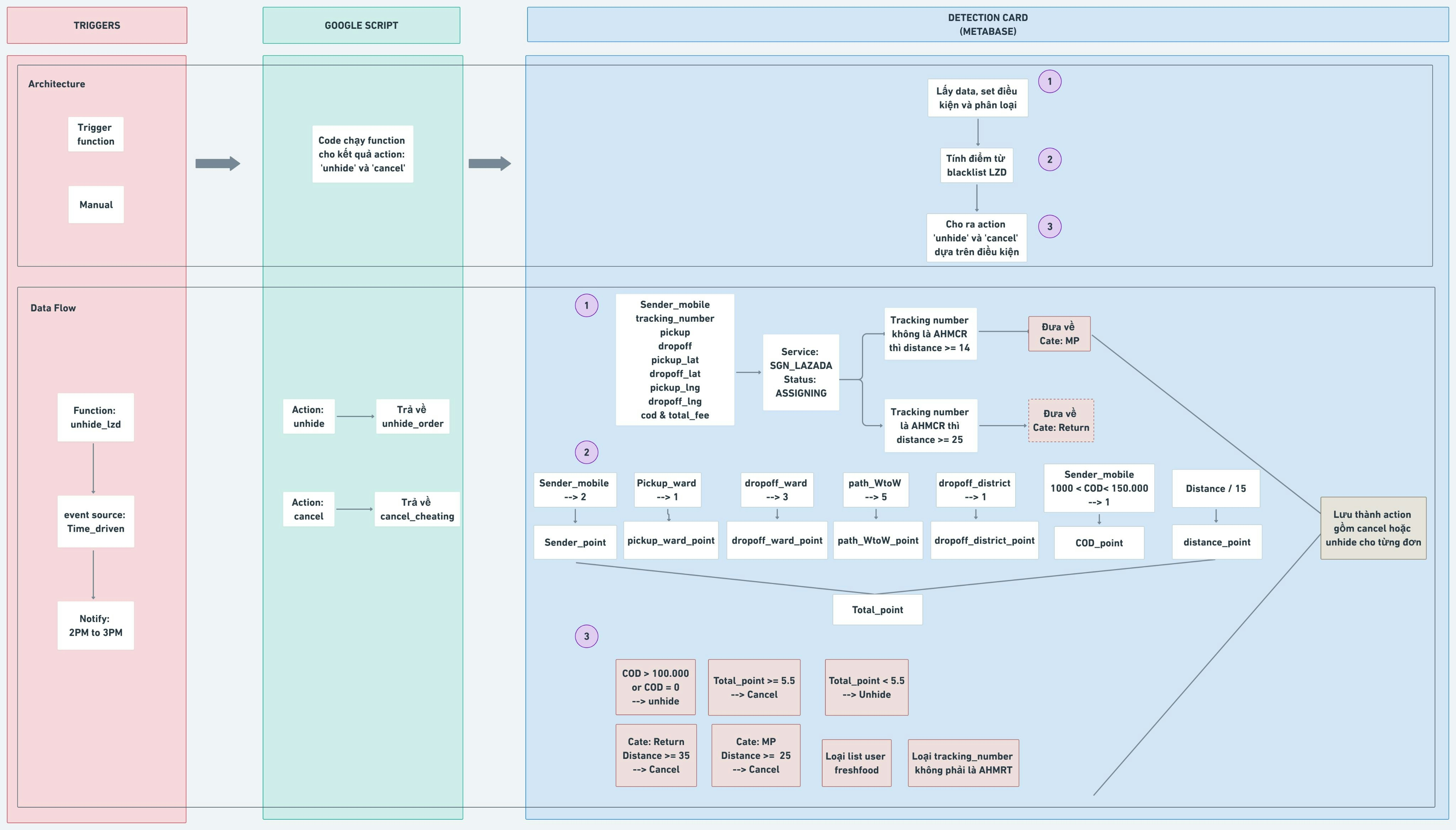Share
Explore# Overall

Trạng thái đơn hàng: ASSIGNING# 1 - Metabase

## Extract data for detection

Viết card tính điểm và phân loại 2 tập Cancel và Unhide
total_pọint = sender_point + pickup_ward_point + dropoff_ward_point + path_wtow_point + dropoff_district_point+distance_point
COD > 100000 hoặc COD = 0 thì unhide
Cate = Return và distance >=35 thì cancel
Cate = MP và distance >= 25 thì cancel
Total_point >= 5.5 thì cancel

`with``stp as (select id`` , to_char(to_timestamp(o.create_time),'YYYY-MM-DD HH24:MI:SS') as create_time`` , path->0->>'name' as sender_name`` , right(path->0->>'mobile',9) as sender_mobile`` , (path->1->>'weight')::numeric as weight `` , (path->1->>'tracking_number') as tracking_number `` , o.distance`` , (o.path->0->>'address') as pickup`` , (path->1->>'address') as dropoff`` , (path->0->>'lat') as pickup_lat `` , (path->0->>'lng') as pickup_lng `` , (path->1->>'lat') as dropoff_lat `` , (path->1->>'lng') as dropoff_lng `` , total_fee`` , coalesce(cast((path->1->>'cod') as numeric),0) as cod`` , supplier_id, supplier_name``from "order" o`` where 1=1`` and order_date >= current_date - interval '0 day'`` and service_id = 'SGN-LAZADA'`` and status = 'ASSIGNING'`` and (Case when`` path->1->>'tracking_number' not like '%AHMCR%' then distance >= 14`` when`` path->1->>'tracking_number' like '%AHMCR%' then distance >= 25 end )``)`
`, stp_location as (``select `` g.id, case when tracking_number like '%AHMCR%' then 'Return' else 'MP' end as cate`` , create_time`` , tracking_number`` , distance`` , sender_name`` , sender_mobile `` , pickup`` , dropoff `` , c2.name_vn as pickup_district `` , c1.name_vn as dropoff_district`` , c4.name_vn||' '||c2.name_vn as pickup_ward`` , c3.name_vn||' '||c1.name_vn as dropoff_ward`` , c4.name_vn||' '||c2.name_vn||'-'||c3.name_vn||' '||c1.name_vn as path_wtow`` , total_fee`` , cod ``from stp g`` left join admin_areas as c1`` on ST_Contains(c1.the_geom, ST_SetSRID(ST_POINT(g.dropoff_lng ::float, g.dropoff_lat ::float),4326))`` left join admin_areas as c2`` on ST_Contains(c2.the_geom, ST_SetSRID(ST_POINT(g.pickup_lng ::float, g.pickup_lat ::float),4326))`` left join admin_areas as c3`` on ST_Contains(c3.the_geom, ST_SetSRID(ST_POINT(g.dropoff_lng ::float, g.dropoff_lat ::float),4326))`` left join admin_areas as c4`` on ST_Contains(c4.the_geom, ST_SetSRID(ST_POINT(g.pickup_lng ::float, g.pickup_lat ::float),4326))`` where 1=1 `` and c1.level = '3' `` and c2.level = '3'`` and c3.level = '4' `` and c4.level = '4'`` )`
`, point as ( select *,`` case when sender_mobile in (select seller_right from custom.lzd_blacklist) then 2 else 0 end as sender_point,`` case when pickup_ward in (select pickup_ward from custom.lzd_blacklist) then 1 else 0 end as pickup_ward_point,`` case when dropoff_ward in (select dropoff_ward from custom.lzd_blacklist) then 3 else 0 end as dropoff_ward_point,`` case when path_wtow in (select path_wtow from custom.lzd_blacklist) then 5 else 0 end as path_wtow_point,`` case when dropoff_district in (select dropoff_district from custom.lzd_blacklist) then 1 else 0 end as dropoff_district_point,`` case when sender_mobile in (select seller_right from custom.lzd_blacklist) and cod between 1000 and 150000 then 1 else 0 end as cod_point,`` (distance/15) as distance_point`` from stp_location )`` ``, total_point as (select sender_point+pickup_ward_point+dropoff_ward_point+path_wtow_point+dropoff_district_point+distance_point as total_point, * from point``order by 1 desc)`
`, fin as (select case ``when cod > 100000 or cod = 0 then 'unhide'``when cate = 'Return' and distance >=35 then 'cancel'``when cate = 'MP' and distance >= 25 then 'cancel'``when total_point >= 5.5 then 'cancel' ``else 'unhide' end as action, * ``from total_point ``where right(sender_mobile,9) not in (select right(mobile,9) from custom.freshfood) -- loại list user FF``order by total_point desc)`
`select * from fin``where true ``and tracking_number not like '%AHMRT%'``[[and action = {{action}}]] order by 1`

# 2 - Google Apps Script

## ⁠Link Script⁠ > Files Unhide order

`function unhide_lzd() {`` var url1 = "https://admin.ahamove.com/public/v1/bi/metabase_card?cardid=21544&action=unhide"`` var response1 = UrlFetchApp.fetch(url1);`` var dataSet = JSON.parse(response1);`` Logger.log(dataSet);`` for (i=0 ; i < dataSet.length; i++)`` {`` var id = dataSet[i].id;`` Logger.log(id);``// for (j = 0 ; j < re.length; j++)``// {`` unhide_order(id);``// }`` }`
` var url2 = "https://admin.ahamove.com/public/v1/bi/metabase_card?cardid=21544&action=cancel"`` var response2 = UrlFetchApp.fetch(url2);`` var dataSet2 = JSON.parse(response2);`` Logger.log(dataSet2);`` for (i=0 ; i < dataSet2.length; i++)`` {`` var id = dataSet2[i].id;`` Logger.log(id);``// for (j = 0 ; j < re.length; j++)``// {`` cancel_cheating(id);``// }`` } `
`}`

# 3 - Setup Triggers

## Auto

Function: Unhide_lzd
Notify daily 2PM to 3PM
Action: unhide > trả về unhide_oder ID
Action: cancel > trả về cancel_cheating ID

## Manual (bị lỗi không tự động triggers)

Chọn function Unhide_order
Bấm Run để chạy trigger thủ công.